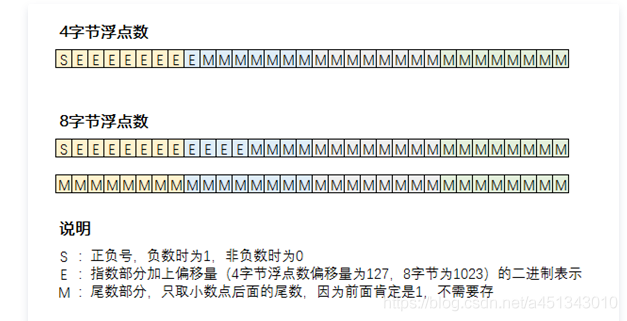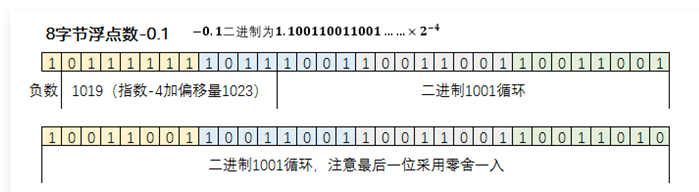# float

2022/02/13

IEEE-754标准表示的二进制浮点数，包含三个部分：符号位、指数部分和尾数部分

## 浮点型结构

• float32（单精度类型，占据4个字节 byte，32个二进制位 bit）
• float64（双精度类型，占据8个字节 byte，64个二进制位 bit）

3.5 11.1 2^0 + 2^0 + 2^-1
10.625 1010.101 2^3 + 2^1 + 2^-1 + 2^-3
0.6 0.10011001… 2^-1 + 2^-4 + 2^-5 + 2^-8 + …

3.5 11.1 1.11 * 2^1
10.625 1010.101 1.010101 * 2^3
0.6 0.10011001… 1.0011001… * 2^-1

• 正负号
• 指数
• 尾数• 字节浮点数的偏移量为 127
• 字节浮点数的偏移量为 1023

4字节浮点数的指数部分为 -7 ，则通常表示为 10000111 1为符号位，代表它是一个负数。

7 表示为 00000111 0为符号位，代表它是一个负数。

-7变成 120 ，二进制表示为 01111000

## 具体事例

### 二进制表示

``````fmt.Printf("%b", math.Float32bits(data))
``````

3 0 10000000 10000000 00000000 0000000 11.0 = 1.1*2^1 指数部分为127+1=128，尾数部分为1
1 0 01111111 00000000 00000000 0000000 1.0 = 1.0*2^0 指数部分为127，尾数部分为0
0.5 0 01111110 00000000 00000000 0000000 0.1 = 1.0*2^-1 指数部分为127-1=126，尾数部分为0
-0.25 1 01111101 00000000 00000000 0000000 -0.01 = -1.0*2^-2 指数部分为127-2=125，尾数部分为0，符号位为1

### 问题描述

`fmt.Println(float64(float32(0.3)) == float64(0.3))`

## 判断浮点数是否相等

### 转换成string类型

``````func Decimal(value float32) string {
value1 := fmt.Sprintf("%.6f", value)
return value1
}
``````
``````func Compare(val1,val2 string) bool {
indexf :=strings.Index(val1,".") + 4
indexs :=strings.Index(val2,".") + 4
if indexs != indexf {
return false
}else {
if val1[0:indexf] == val2[0:indexs]{
return true
}else {
return false
}
}
}
``````

### 精度判断

``````package main

import (
"fmt"
"math"
)
const MIN = 0.000001
// MIN 为用户自定义的比较精度
func IsEqual(f1, f2 float64) bool {
if f1>f2{
return math.Dim(f1, f2) < MIN
}else{
return math.Dim(f2, f1) < MIN
}
}
func main() {
a := 0.9
b := 1.0

if IsEqual(a, b) {
fmt.Println("a==b")
}else{
fmt.Println("a!=b")
}
}
``````